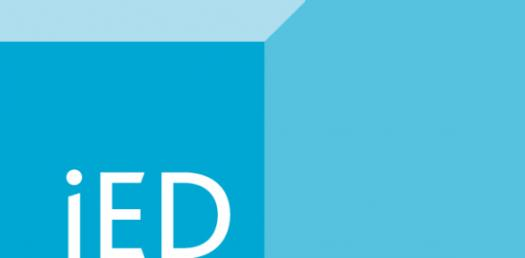IED: Institution Of Engineering Designers Exam Questions! Trivia Quiz

1 Question | Total Attempts: 489Settings.

• 1.
An innovation is ....
• A.

A new product, system, or process that has never existed before

• B.

An improvement of an existing technological product, process, or system

• C.

An idea to solve a problem

• D.

Method of determining how a machine functions

• 2.
A design brief is defined as ....
• A.

An iterative decision-making process that produces a solution to a problem

• B.

A technique for solving problems

• C.

A written plan that identifies a problem to be solved, its criteria, and its constraints

• D.

The systematic study of materials and sources in order to establish facts and reach new conclusions

• 3.
___ is used to formally document, in chronological order, all work associated with a specific design project.
• A.

Portfolio

• B.

Engineering log

• C.

Engineering notebook

• D.

Design brief

• 4.
A method of representing 3-D objects on a plane having only width and height is a ___.
• A.

Multi-view drawing

• B.

Orthographic projection

• C.

Perspective sketch

• D.

Oblique sketch

• 5.
A ___ is an oblique pictorial where the depth is represented as half scale but the width and height are full scale.
• A.

Cavalier pictorial

• B.

Cabinet pictorial

• C.

Orthographic projection

• D.

Perspective sketch

• 6.
According to the drawing below, what is the maximum diameter for hole H?
• A.

.500

• B.

.520

• C.

.502

• D.

.250

• 7.
Which of the following disciplines involves the complex analysis, design, and development of solutions to technical problems?
• A.

Mathematics

• B.

Engineering

• C.

Science

• D.

Medicine

• 8.
Which of the following is a design element?
• A.

Emphasis

• B.

Balance

• C.

Rhythm

• D.

Color

• 9.
During which step of the design process would a design brief be written?
• A.

Problem Identification

• B.

Design Analysis

• C.

Development and Implementation

• D.

Conceptualization

• 10.
A designer will often keep ________________ of his or her best work to show off to prospective clients.
• A.

A design brief

• B.

A flash drive

• C.

A portfolio

• D.

N engineer's notebook

• 11.
The shape pictured on the right is called ______________.
• A.

A circle

• B.

An ellipse

• C.

A spline

• D.

A parallelogram

• 12.
Of the pictorial sketches pictured below, which one is a two-point perspective?
• A.

Figure 1

• B.

Figure 2

• C.

Figure 3

• D.

Figure 4

• 13.
___________ is a type of drawing that is done freehand and without the use of drawing tools.
• A.

Computer-aided Design

• B.

Sketching

• C.

Prototyping

• D.

Isometric

• 14.
The image below is referred to as a ___.
• A.

Pie chart

• B.

Histogram

• C.

Gantt chart

• D.

Chart of Wedges

• 15.
The image below is referred to as a ___.
• A.

Pie chart

• B.

Histogram

• C.

Gantt chart

• D.

Line graph

• 16.
What type of full-scale physical model would an engineer use to demonstrate how a design functions?
• A.

Prototype

• B.

Inventor Assembly

• C.

Conceptual Model

• D.

Appearance Model

• 17.
A full-size clay model of a new car body design would be considered a ____________.
• A.

Prototype

• B.

Mock-up

• C.

Conceptual model

• D.

Mathematical model

• 18.
What is the total surface area of a 3” cube?
• A.

54 square inches

• B.

27 square inches

• C.

36 square inches

• D.

45 square inches

• 19.
The list below shows the amount of time that it took for four students to solve a puzzle. What was the average solution time? • Amy – 5 minutes 0 seconds • Bob – 4 minutes 32 seconds • Teresa – 3 minutes 21 seconds • Jim – 2 minutes 58 seconds
• A.

2 minutes, 58 seconds

• B.

3 minutes, 58 seconds

• C.

5 minutes, 0 seconds

• D.

15 minutes, 51 seconds

• 20.
What 3D solid modeling feature would be used to establish a two-dimensional drawing surface that is tangent to the outside of a sphere?
• A.

Sketch plane

• B.

Revolve

• C.

Origin

• D.

Work plane

• 21.
A piece of plastic has a density of 75 g/L. What is the density in pounds per cubic foot?
• A.

4.68 lb / cubic foot

• B.

165.4 lb / cubic foot

• C.

.165 lb / cubic foot

• D.

.00584 lb / cubic foot

• 22.
When calculating the density of an irregularly shaped object, scientists and engineers can use ___ to determine the volume of the object.
• A.

Liquid displacement

• B.

A balance

• C.

A measuring cup

• D.

A tape measure

• 23.
A drawing which contains views of an object projected onto two or more orthographic planes is a(n) ___.
• A.

Cabinet pictorial

• B.

Cavalier pictorial

• C.

Oblique pictorial

• D.

Multi-view drawing

• 24.
A rope measures 9.25 yards. What is the measurement in feet?
• A.

27.75 ft

• B.

27 ft

• C.

27.5 ft

• D.

9 ft

• 25.
A metal rod needs to be cut for a machine in Greenville. The rod length should be 67.5 cm, but your equipment only measures in American standard units. What measurement should you set on your manufacturing computer to get the correct length?
• A.

2 ft

• B.

171.45 in

• C.

26.57 in

• D.

100 in

Related TopicsBack to top